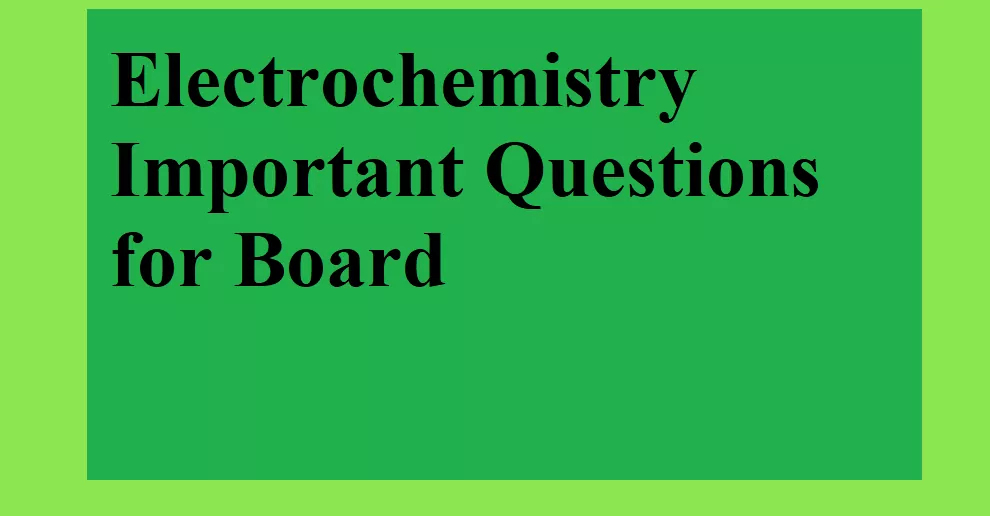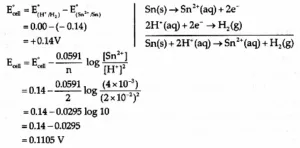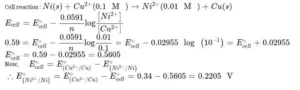# Electrochemistry Important Questions for BoardElectrochemistry Important questions for Board

Que 1. i)  Define the molar conductivity of a solution and explain how molar conductivity changes with a change in concentration of solution for a weak and strong electrolyte.
ii) The resistance of a conductivity cell containing 0.001M KCl solution at 298 K is 1500Ω. What is the cell constant, if the conductivity of 0.01 M KCl solution at 298 K is 0.146 × 10–3 S cm–1?
Ans 1. i) Molar conductivity – it is defined as the conductance of the solution which contains one mole of an electrolyte such that the entire solution is in between two electrodes kept one centimeter apart sharing unit area of cross-section
(ii) Given conductivity K = 0.146 × 10–3 S cm–1
Cell constant G = k (R) = 0.146 × 10–3 × 1500 = 0.219 cm–1

Que 2. How much electricity in terms of Faraday is required to produce 100g of Ca from molten CaCl2?
Ans 2. We have to note that one mole of electron charge is equivalent to one Faraday.
Given reaction,
CaCl2​→ Ca2+ + 2Cl
Ca is undergoing oxidation i.e, Ca2+ + 2e → Ca
We can observe that 40g of Ca takes 2e− charge,
1 mole of Ca(40g) = 2F
Now, 100g of Ca = 5 F

Que 3. Name the solid substance produced, during the discharge of the lead-storage battery.

Electrochemistry Important questions for Board

Que 4. (i) Write the formulation for galvanic cell in which the reaction
Cu(s) + 2 Ag+ (aq) → Cu2+(aq) + 2Ag(s) takes place, identify the cathode and the anode reaction in it.
ii) Write Nernst equation. Calculate the emf of the following cell:
Sn(s) / Sn2+ (0.004M) // H+ (0.020 M) / H2(g), Pt(s) (given E0Sn2+/Sn = + 0.14 V).
Ans 4. (i) Cu(s) → Cu2+ (aq) + 2e (at anode)
2 Ag+ (aq) + 2e → 2 Ag(s)
Cu/Cu++ || Ag+|Ag
Cu(s) + 2 Ag+ (aq) → Cu2+ (aq) + 2 Ag(S)
(ii).Que 5. Give an example of a fuel cell and write the cathode and anode reaction for it.
Ans 5. The galvanic cell that is designed to convert the energy of combustion of fuel like hydrogen, methane, methanol directly into electrical energy is called a fuel cell.
Cathode reaction: O2 (g) + 2 H2O (l) + 4e → 4 OH (aq)
Anode reaction: 2 H2 (g) + 4 OH (aq) → 4 H2O (l) + 4e
Overall reaction: 2 H2 (g) + O2 (g) → 2H2O(l)

Que 6. The resistance of conductivity cell filled with 0.1 M KCl solution is 100 Ohm. If the resistance of the same cell when filled with 0.02 M KCl solution is 520 ohm, calculate the conductivity and molar conductivity of 0.02 M KCl solution. Conductivity of 0.1 M KCl solution is 1.29 m–1
Ans 6. i) Cell constant G = conductivity (resistance)
= 1.29 S m–1 (100Ω) = 129 m–1 or 1.29cm–1
ii) Conductivity of 0.02 M KCl solution (k) = cell constant/resistance = 1.29 cm–1/520Ω = 2.48 × 10–3 S cm–1
iii) Molar conductivity = k × 1000\M = 2.48 x 10–3 x 1000/0.02 = 124 S cm2 mol–1

Electrochemistry Important questions for Board

Que 7. Calculate the standard electrode potential of Ni2+/Ni electrode if emf of the Ni2+/Ni(0.01) // Cu2+(0.1)/Cu (s) electrochemical cell is 0.59V. Given E0 = 0.34 V
Ans 7.Que 8. What type of battery is the lead storage battery? Write the anode and the cathode reaction and the overall reaction occurring when current is drawn from it (reaction during discharge of it)
Ans 8. Lead storage battery is the secondary cell. So it can be recharged by passing direct current through it.
Discharge reaction –
At anode: Pb(s) + SO42– (aq) → PbSO4 (s) +2e
At cathode: PbO2(s) + SO42– (aq) + 4H+ (aq) + 2e → 2PbSO4 (aq) + 2H2O(l)
Overall solution: Pb + PbO2 + 4H+ + SO42– (aq) → 2PbSO4 + 2H2O

Que 9. How many moles of mercury will be produced by electrolyzing 1.0 M Hg(NO3)2 solution with a current of 2.00 A for three hours ? Molar mass of Hg(NO3)2 = 200.6 g/mol
Ans 9. Current = 2A
Time = 3h = 3 × 60 × 60s = 10800 s
Quantity of charge passed (Q) = I × t = 2.0A × 10800 = 21600 AS = 21600 C
Hg(NO3)2 → Hg2+ + 2NO3
Hg+ + 2e→ Hg(s)
2 × 96500 C  1mol
When 2 × 96500 C is passed, mercury deposited = 1 mol
When 1 C is passed, mercury deposited = 1/ 2 × 96500 = 1/ 193000
When 21600 C is passed, mercury deposited = 21600/193000 = 0.112 mol

Electrochemistry Important questions for Board

Que 10. Why mercury cell gives constant voltage throughout its life?
Ans 10. As the overall reaction does not involve any ion in a solution whose conc. can change during its life.

Que 11. What is the effect of dilution of concentration on specific conductance?
Ans 11. Specific conductance decreases with dilution because it is the conduction power of ion present in a unit volume of solution and the number of ion in unit volume decreases on dilution. Specific conductance increases with concentration.

Que 12. The following electrochemical cell has been set up
Pt (1) | Fe3+, Fe2+ (a=1) || Ce4+, Ce3+ (a=1) | Pt (2)
Predict the direction of flow of current (given : E0Ce4+, Ce3+ = 1.61 V; E0 Fe3+, Fe2+  = 0.77 V).
Ans 12. The electrode reactions:
Anode: Fe2+ → Fe3+
Cathode: Ce4+ + e → Ce3+
Cell Reaction: Fe2+ + Ce4+ → Fe3+ + Ce3+
Cell potential is E0Cell = E0Cathode – E0Anode = 1.61 – 0.77 = 0.84 V
Since ECell is positive, the cell reaction is spontaneous. The current will flow from Pt(2) to Pt (1) – Flow of e from Anode to Cathode; by convention, direction of current flow from Cathode to Anode. With the passage of time, EMF of cell decrease and so does the current flow.

Electrochemistry Important questions for Board

Que 13. Why is it not possible to measure single electrode potential?
Ans 13. Because the half cell containing a single electrode cannot exist independently, as charge cannot flow on its own in a single electrode.

Que 14. Define corrosion. What is the chemical formula of rust?
Ans 14. Corrosion is the slow eating away of the surface of the metal due to the attack of atmospheric gases. Fe2O3.xH2O.

Que 15. A solution of CuSO4 is electrolyzed for 10 minutes with a current of 1.5 amperes. What is the mass of copper deposited at the cathode?
Ans 15. Current (I) = 1.5 Ampere
Time = 10 × 60s = 600s
Q = I × t = 1.5 × 600 = 900 C
Cu2+ + 2e → Cu (s)
2F amount of electricity deposit copper = 63.5 g
900 C amount of electricity deposit copper = (63.5 × 900)/(2 × 96500)
= 0.296 g

Electrochemistry Important questions for Board